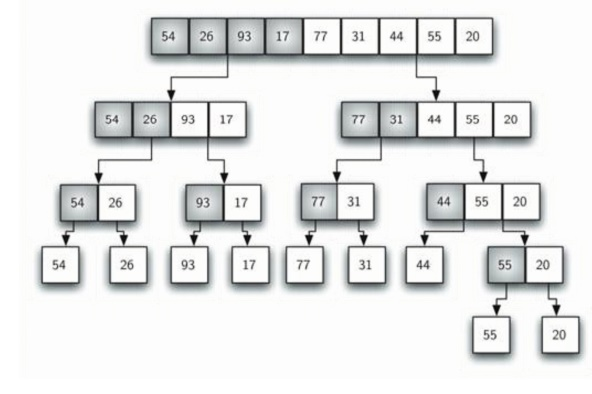# Merge Sort

The first algorithm we will study is the merge sort. Merge sort is a recursive algorithm that continually splits a list in half.

Merge Sort

We now turn our attention to using a divide and conquer strategy as a way to improve the performance of sorting algorithms. The first algorithm we will study is the merge sort. Merge sort is a recursive algorithm that continually splits a list in half. If the list is empty or has one item, it is sorted by definition (the base case). If the list has more than one item, we split the list and recursively invoke a merge sort on both halves. Once the two halves are sorted, the fundamental operation, called a merge, is performed. Merging is the process of taking two smaller sorted lists and combining them together into a single, sorted, new list. Figure below shows our familiar example list as it is being split by mergeSort. figure 4shows the simple lists, now sorted, as they are merged back together. The mergeSort function shown in ActiveCode 6 begins by asking the base case question. If the length of the list is less than or equal to one, then we already have a sorted list and no more processing is necessary. If, on the other hand, the length is greater than one, then we use the Python slice operation to extract the left and right halves. It is important to note that the list may not have an even number of items. That does not matter, as the lengths will differ by at most one.MERGE SORT for PSEUDOCODE:

def mergeSort(alist):

print("Splitting ",alist)

if len(alist)>1:

mid = len(alist)//2

lefthalf = alist[:mid]

righthalf = alist[mid:]

mergeSort(lefthalf)

mergeSort(righthalf)

i=0

j=0

k=0

while i<len(lefthalf) and j<len(righthalf):

if lefthalf[i]<righthalf[j]: alist[k]=righthalf[j]

j=j+1

k=k+1

while i<len(lefthalf): alist[k]=lefthalf[i] i=i+1

k=k+1

while j<len(righthalf): alist[k]=righthalf[j] j=j+1

k=k+1 print("Merging ",alist)

alist = [54,26,93,17,77,31,44,55,20]

mergeSort(alist)

print(alist)

Study Material, Lecturing Notes, Assignment, Reference, Wiki description explanation, brief detail
Object Oriented Programming and Data Structure : Sorting And Searching : Merge Sort |